# Where Does Energy On Our Planet Come From?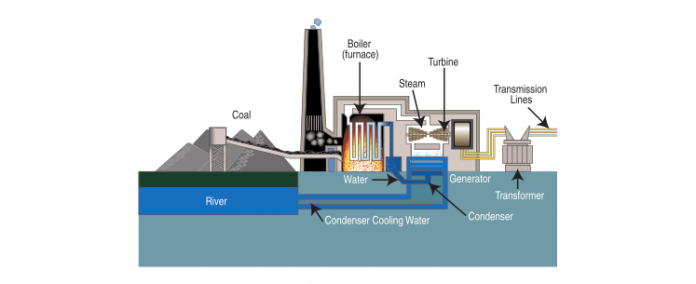There are three primary sources of material amounts of energy on Earth. The word material means an amount of energy greater than 2 % of total worldwide energy demand combining all sectors of transportation, industrial, commercial residential, agricultural, potable water production and its movement. Energy sources such as thermal piles, batteries and the like are not considered material. These are: Energy from the Sun. The most prevalent form of useful energy today comes from the Sun in the form of hydrocarbon based fossil fuels like petroleum, coal, and natural gas. The energy is transformed to do useful work by chemical reactions which generate heat when these fuels are combined with oxygen and burned. Fossil fuels were created through photosynthesis in combination with the processes of plant and animal life from the energy provided by the Sun over the last 400 million years of the Earth’s existence. Over a 400 year period, man will have depleted economically viable fossil fuel reserves. Vastly smaller amounts of energy generated by the sun can be produced through solar energy process such as photo voltaic and concentrated solar. Additionally, wind and river water movement can be used to generate electricity which is a form of energy. Both wind and the movement of water in the Earth’s vast system of rivers occur because of solar energy. Ocean tidal movement is created by solar system gravitational forces. Ocean currents can be induced by solar energy. Both tidal and ocean current hydrokinetic are not practical for material energy collection. Energy from nuclear fission. In nuclear fission, energy is released by the atomic reaction of splitting heavy elements such as uranium, element 92, isotope 235, or thorium element number 90, isotope 232. Thorium 232 is relatively abundant on Earth. Uranium 235 is far less abundant. It is estimated that there is enough useable thorium 232 on the Earth to provide the electricity energy consumed by the United States for the next 1,000 years based on current energy use. However, the nuclear process of splitting atoms results in many problems such as nuclear radiation, long term dangerous radioactive waste, possible explosive chain reactions, melt downs of fuel cores, and basic fissile fuel sources having military uses in weapons and the related threats. Contrary to a common believe however, a nuclear reactor cannot explode in the sense of an atomic bomb nor can it melt ground beneath it and drill down. Energy from atomic fusion. Fusion is what powers the Sun and all the stars. Energy is produced by the process of nuclear fusion when two light element atoms are fused into a slightly heavier atom along with a corresponding large release of energy. An example is the well-known and well understood fusion reaction of D (Hydrogen 2) + T (Hydrogen 3) > He4 (Helium) + 17.6 MeV (energy units) + n (neutron.) This is the fundamental process resulting from the big bang or creation of the universe event. This is also the fundamental process of the Sun. As we understand fusion in the future, other fusion reactions can make use of helium, boron and sub-atomic particles such as protons. The Earth has enough of these abundant materials to power the entire world’s energy needs literally for eternity. Fusion is more difficult to accomplish in a controlled environment than fission. Fusion is a creation process whereas fission is a destruction process. In fission once a critical mass of heavy element fissionable material is assembled, it destroys itself and decays into highly radioactive elements of lower atomic mass. Fusion, on the other hand requires energy to be pumped into the system to a point where the net energy gain resulting from the bringing together of light atoms is considerably greater than the energy pumped in. Based on the above analysis we can see that all material energy forms on Earth come from atomic reactions; either the sun or its fusion counterpart on Earth or from nuclear fission.

#### What is energy?

In physics, energy is defined as the capacity for doing work. It may exist in potential, kinetic, thermal, electrical, chemical, atomic, or various other forms. There are, heat and work—i.e., energy in the process of transfer from one body to another. After it has been transferred, energy is always designated according to its nature. Hence, heat transferred may become thermal energy, while work done may manifest itself in the form of mechanical energy. All forms of energy are associated with motion. For example, any given body has kinetic energy if it is in motion. A tensioned device such as a bow, spring, or water storage reservoir, though at rest, has the potential for creating motion; it contains potential energy because of its configuration. Similarly, atomic energy is potential energy because it results from the configuration of subatomic particles in the nucleus of an atom. Thermal or heat energy comes from the vibration of atoms as energy is absorbed through emitted photons from electromagnetic radiation. Energy can be converted from one form to another in various ways. Usable mechanical or electrical energy is, for instance, produced by many kinds of devices, including fuel-burning heat engines, generators, batteries, fuel cells, and magnetohydrodynamics systems. Energy can be neither created nor destroyed but can be converted from one form to another and energy and mass are different manifestations of the same thing and each can be converted to the other form in the proportion of E=mc2

#### Conservation of Mass and Energy Law

The Law of Conservation of Energy states that energy cannot be created or destroyed, but can change its form. The total quantity of matter and energy available in the universe is a fixed amount and never any more or less. The law of conservation of mass or matter, also known as the Lomonosov-Lavoisier law, states that the mass of substances in a closed system will remain constant, no matter what processes are acting inside the system. It is a different way of stating that though matter may change form, it can be neither created nor destroyed. The mass of the reactants must always equal the mass of the products. This law works fine for anything that is not approaching the speed of light; at high speeds, mass begins transforming to energy (for which reason, we now have the Law of Conservation of Mass and Energy). However, this means that in most situations the law of conservation of mass can be assumed valid using standard Newtonian based classical physics. However the mass–energy relation of E=mc2 states that the universal proportionality factor between equivalent amounts of energy and mass is equal to the velocity of light squared. This also serves to convert units of mass to units of energy, no matter what system of measurement units is used. This law was first formulated by Antoine Lavoisier in 1789, but Mikhail Lomonosov in 1748 had also expressed similar ideas earlier. It was the key to making chemistry into a real science instead of an offshoot of alchemy; prior to this, buoyancy of gases made it difficult to determine before and after measurements of weight. In nuclear reactions and in very large astronomical objects, this law becomes questionable. After this, the ideas of chemical elements, process of fire and oxidation, and many other basic chemical principles could be understood. One of the first conservation laws to be discovered was the conservation of mass (or matter). Suppose that you combine a very accurately weighed amount of iron (Fe) and sulfur (S) with each other. The product of that reaction is a compound known as iron sulfide or FeS. If you also weigh very accurately the amount of iron sulfide formed in that reaction, you will discover a simple relationship: The weight of the beginning materials (iron plus sulfur) is exactly equal to the weight of the product or products of the reaction (iron sulfide). This statement is one way to express the law of conservation of mass. A more formal definition of the law is that mass (or matter) cannot be created or destroyed in a chemical reaction. A similar law exists for energy. When you turn on an electric heater, electrical energy is converted to heat energy. If you measure the amount of electricity supplied to the heater and the amount of heat produced by the heater, you will find the amounts are equal. In other words, energy is conserved in the heater. It may take various forms, such as electrical energy, heat, magnetism, or kinetic energy (the energy of an object due to its motion), but the relationship is always the same: The amount of energy used to initiate a change is the same as the amount of energy detected at the end of the change. In other words, energy cannot be created or destroyed in a physical or chemical change. This statement summarizes the law of conservation of energy. At one time, scientists thought that the law of conservation of mass and the law of conservation of energy were two distinct laws. In the early part of the twentieth century, Albert Einstein (1879–1955) demonstrated that matter and energy are two forms of the same thing. He showed that matter can change into energy and that energy can change into matter. Einstein’s discovery required a restatement of the laws of conservation of mass and energy. In some instances, a tiny bit of matter can be created or destroyed in a change. The quantity is too small to be measured by ordinary instruments, but it still amounts to something. Similarly, a small amount of energy can be created or destroyed in a change. But, the total amount of matter PLUS energy before and after a change still remains constant. This statement is now accepted as the law of conservation of mass and energy. Einstein went on to express the relationship between energy and mass as E=MC2, where E is energy, M is mass and C is the velocity of light (299.8 million meters per second) Squared or 8.98 X 1016. Because C2 is such a big number, this means a very small amount of mass can be converted into a huge amount of energy. This is why fission and fusion produce so much power from so little “fuel.” Examples of the law of conservation of mass and energy are common in everyday life. The manufacturer of an electric heater can tell consumers how much heat will be produced by a given model of heater. The amount of heat produced is determined by the amount of electrical current that goes into the heater. Similarly, the amount of gasoline that can be formed in the breakdown of petroleum can be calculated by the amount of petroleum used in the process. And the amount of nuclear energy produced by a nuclear power plant can be calculated by the amount of uranium-235 used in the plant. Calculations such as these are never quite as simple as they sound. We think of an electric light bulb, for example, as a way of changing electrical energy into light. Yet, more than 90 percent of that electricity is actually converted to heat. (Baby chicks are kept warm by the heat of light bulbs.) Still, the conservation law holds true. The total amount of energy produced in a light bulb (heat plus light) is equal to the total amount of energy put into the bulb in the form of electricity. In an automobile internal combustion engine, heat is produced by the combustion reaction of gasoline and oxygen which pushes a piston down when the mixture of oxygen and fuel rapidly expands which, in turn, is coupled to a crank shaft which turns and is coupled to the wheels. Modern gasoline engines have a maximum thermal efficiency of about 25% to 30% when used to power a car. In other words, even when the engine is operating at its point of maximum thermal efficiency, of the total heat energy released by the gasoline consumed, about 70-75% is rejected as heat without being turned into useful work, i.e. turning the crankshaft. Approximately half of this rejected heat is carried away by the exhaust gases, and half passes through the cylinder walls or cylinder head into the engine cooling system, and is passed to the atmosphere via the cooling system radiator. Some of the work generated is also lost as friction, noise, air turbulence, and work used to turn engine equipment and appliances such as water and oil pumps and the electrical generator, leaving only about 25-30% of the energy released by the fuel consumed available to move the vehicle. Today, fission, coal, natural gas and oil fired electricity plants work by heating water (or in some cases salts) to its vapor point and using that heat energy to turn an electrical generator motor.

#### Where does today’s energy to convert to electricity come from?

 Energy Source Quads① Percent of total Coal 16.4 42.71% Natural Gas 8.37 21.79% Nuclear 8.33 21.69% Hydro 2.44 6.35% Wind 1.73 4.51% Biomass 0.507 1.32% Petroleum 0.294 0.76% Solar 0.17 0.443% Geothermal 0.159 0.414%
① 1 Quad = 1.055 X 1018 joules. The above table is based on the following (click for the full sized image):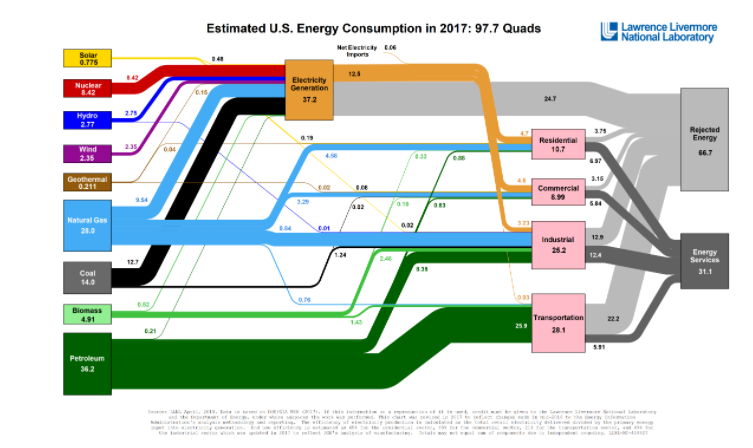February 2016 EIA 0035 Monthly Energy Review

#### How power is generated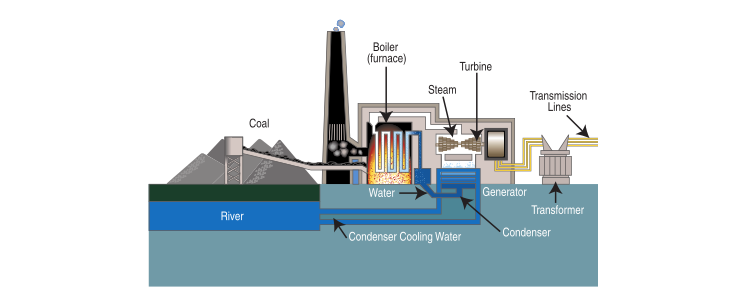Coal fired power plant schematic

Electricity is actually the flow or movement of electrons through a material. An electron is a sub-atomic particle. Electric generating plants typically produce electricity using magnetic induction & conduction. This happens when a large number of conductive wires are spun around inside a magnetic field, causing electrons to move in those wires thereby generating electricity.

In a generating plant, the potential energy of various types of fuels such as coal, natural gas, oil, nuclear and concentrated solar energy is converted into mechanical energy using heat energy to produce the mechanical energy. This mechanical energy is used to turn fan-like blades inside a turbine. These blades are attached to a pole-like shaft. When the blades inside the turbine begin to turn, the shaft begins to turn. This causes wires located inside a magnetic field within the generator to turn. The resulting flow of electrons is electricity. More or less electricity can be created by varying certain factors including: the type of materials used in the wire, the speed at which the turbine rotates, the size of the magnetic field, and the number of wire coils inside the magnetic field, among others.

Wires coming from the generator are used to conduct the flow of electricity out to a neighboring switchyard, where the electricity is “stepped up” or raised to a much higher voltage using transformers so that it can be sent to customers over the transmission and distribution grid.

Steam-electric plants produce electricity by using heat energy to turn water into steam. The highly pressurized steam then travels through pipes to the blades in the turbine. When the steam hits the turbine, it causes the blades to spin.

Hydroelectric generating facilities use mechanical energy from the movement of water to cause the blades in the turbine to turn.

In a steam-electric solar generating facility, heat from the sun’s rays is used to create the steam that is needed to rotate the turbine.

The generator portion of the plants is virtually the same regardless if it is driven by water at a dam, coal, oil, natural gas, nuclear, or concentrated solar.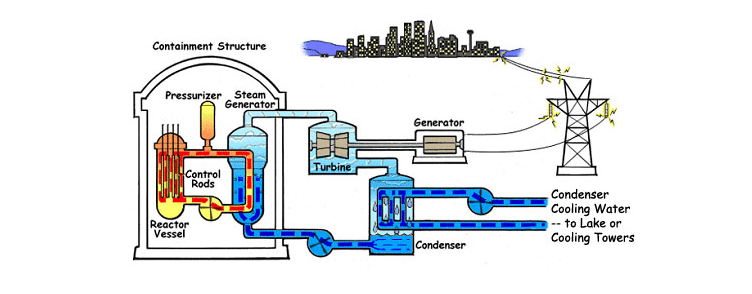Nuclear powered plant schematic

#### Comparison of Fusion Power Density

Energy density is the amount of energy stored in a given system or region of space per unit volume. Specific energy is the amount of energy stored per unit mass (weight.) Only the useful or extractable energy is measured. It is useful to compare the energy densities of various energy sources. At the top of the list is fusion followed by nuclear fission and then hydrocarbon fuels derived from petroleum, coal and natural gas. At the bottom of the list are batteries which either generate energy or store energy as well as “renewable energy” such as solar. 1 Kg of Deuterium fused with 1.5 Kg of Tritium can produce 87.4 GWH of electricity Here are the underlying calculations supporting the statement above: The energy released by fusion of 1 atom of Deuterium with 1 atom of Tritium is 17.6 Mev = 2.8 X 10-12 Joules. The energy liberated by the fusion of 1 Kg of Deuterium with 1.5 Kg of Tritium is 2.8 X 10-12 X 2.99 X 1026 = 8.3 X 1014 Joules = (8.3 X 1014 ) / (3.6 X 10-12 ) = 230 GWHours. This energy is released as heat. A conventional steam turbine power plant with an efficiency of 38%, would produce 87.4GWH of electricity 1 Deuterium is a naturally occurring isotope of hydrogen readily available from sea water. 2 Tritium is produced in the fusion reactor from Lithium as part of the fuel cycle and energy exchange process. Lithium is an abundant naturally occurring element.

#### Comparison of conventional fuel energy density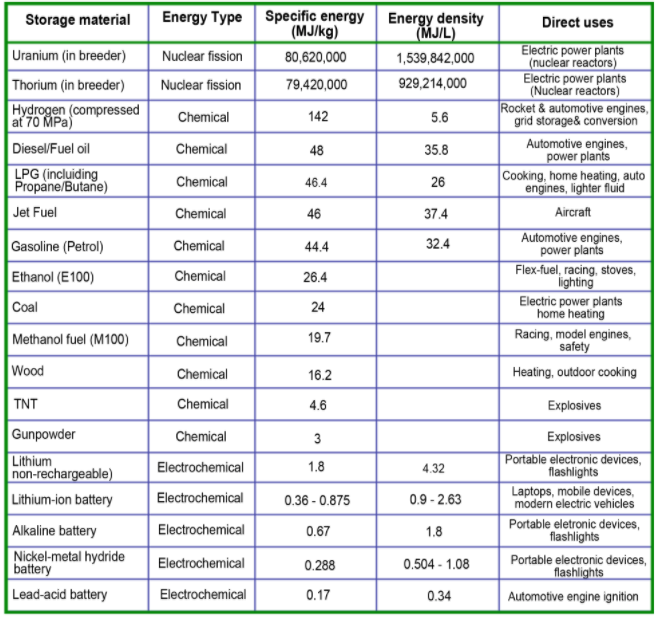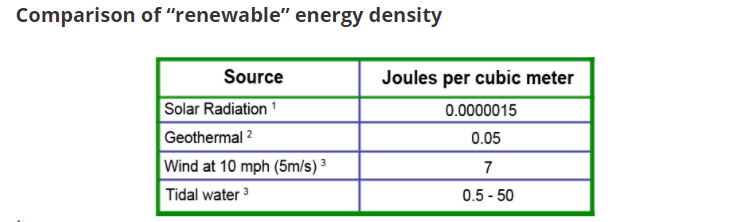1 How much solar power per cubic meter is there? The volume of the space between a one-meter-square patch on Earth and the center of our orbit around the sun is 50 billion cubic meters (the earth is 150 billion meters from the sun, or 4,000 earth circumferences). Dividing the usable 100 watts per square meter by this volume, yields two-billionths of a watt per cubic meter. Sunlight takes about eight minutes(499 seconds) to reach the earth. Multiplying 499 seconds by twenty-six billionths of a W/m3 reveals that solar radiation has an energy density of 1.5 microjoules per cubic meter (1.5 x 10-6 J/m3). 2 The only way to extract thermal energy from the atmosphere is to construct an insulated pipe between it and a reservoir at lower temperature (preferably a much lower one). This is how geothermal heat pumps work. Typical ground temperature is 52F (284 K). On a 90F day, such a system has a peak efficiency of 7%, and a power density of only 0.05 mW/m3 (Stopa and Wojnarowski 2006): typical surface power fluxes for geothermal wells are on the order of 50 mW/m2 and have typical depths of 1 km. To find the energy density, a characteristic time must be included. The time used should be that of the time required for water being pumped into the ground to circulate through the system once. This number is on the order of ten days (Sanjuan et al. 2006). The resulting energy density is 0.05 J/m3, or roughly two to three orders of magnitude lower than wind or waves. 3 Wind is driven by changes in weather patterns, which in turn are driven by thermal gradients. Tides are driven by fluctuations in gravity caused by lunar revolutions. The energy densities of wind and water systems are proportional to the mass, m, moving through them, and the square of the speed, v, of this mass, or ½mv2. At sea level, air with a density of about one kilogram per cubic meter moving at five meters per second (ten miles per hour) has a kinetic energy of 12.5 joules per cubic meter. Applying Betz’s Law, which limits efficiency to 59% (Betz 1926), yields about seven joules per cubic meter. Thus, wind energy on a moderately windy day is over a million times more energy-dense than solar energy. There are two prevalent mechanisms for extracting tidal energy. In one system, barrages move up and down, extracting energy with the rise and fall of the tides. On the second type strategy, tidal stream systems act more like underwater wind turbines, extracting energy from tidal waters as they move past. As with wind, the energy of a moving volume of water is also ½mv2. Tidal systems have the advantage over wind systems in that water is approximately one thousand times denser than air. Their disadvantage lies in generally low tidal velocities of only ten centimeters per second to one meter per second. Thus, a cubic meter of water, with a mass of about 1000 kg, yields an energy density of about five joules per cubic meter for slow water1 and five hundred joules per cubic meter for fast water2. These are also subject to Betz’s law and represent only peak values, so the average energy densities are closer to one-half of a joule per cubic meter to fifty joules per cubic meter, or about the same as wind. 1 kinetic energy (tidal low velocity) = ½ mv2 = ½ · 1000 kg · (0.1 m/s)2 = 5 joules. 2 kinetic energy (tidal high velocity) = ½ mv2 = ½ · 1000 kg · (1 m/s)2 = 500 joules. Originally published on ClimateCite.com